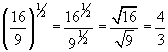# ?

Key Terms

o         Square root

o         Perfect square

o         Cube root

Objectives

o         Understand the meaning of a radical symbol

o         Be able to calculate square roots as well as simple higher-order roots

o         Relate roots to fractional exponents

o         Apply the rules of exponents to derive rules for roots of products and quotients

We will review the meaning of fractional exponents and relate them to radicals. A radical is a symbol that represents a particular root of a number. This symbol is shown below.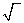Although this symbol looks similar to what is used in long division, a radical is different and has a vastly different meaning. The radical, by itself, signifies a square root. The square root of a number n is written as follows.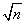The square root of n is defined as another number r such that the square (second power) of r is equal to n.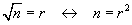Let's take a look at some specific examples to illustrate this somewhat obscure relationship. Consider, for instance, the number 4. Clearly,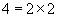, and thus, the square root of 4 is 2. Symbolically,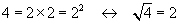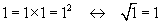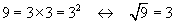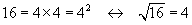The examples above (1, 4, 9, and 16) are called perfect squares, because their square roots are integers. Fractional values also have square roots, however.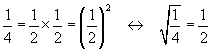In addition, we can calculate the square root of zero.

Interested in learning more? Why not take an online Pre-Algebra course?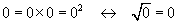Thus, we can calculate the square root of any number greater than or equal to zero; we cannot, however, calculate the square root of a negative number. Note again that the square root of a number n is some number r such that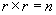. If n is negative and we want to find the square root of n (or), we need to find some number r such that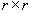is negative. But we know that the product of two negative numbers is positive, and the product of two positive numbers is also positive, as the examples below show.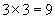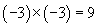Also, the product of zero and zero is zero, so we are left unable to find a number whose square (second power, or the product of the number and itself) is negative. Thus, we consider the square root of a negative number to be undefined. (Actually, an entire branch of mathematics is dedicated to studying square roots of negative numbers; but it is an actual field of study with numerous practical applications in physics and engineering, for instance.)

Practice Problem: Evaluate each square root.

a.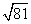b.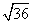c.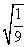d.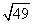Solution: In each case, determine the number n that, if squared (raised to the second power), would equal the number under the radical. None of these requires a calculator, although you may find that a calculator helps you to check your answer.

a.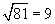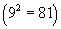b.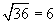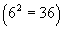c.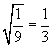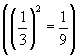d.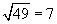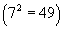Generally, evaluating square roots requires a calculator. Long ("by hand") methods for calculating a square root do exist, however, although they can be tedious. Furthermore, the square roots of many numbers (including integers that are not perfect squares) are irrational. Thus, calculators (including computers) and long methods of calculating the square root can only give approximate results; the actual numbers have an infinite number of decimal places and cannot be written as a fraction with integers in the numerator and denominator. Thus, in the interest of exact math, leaving the numbers in radical form (instead of writing an approximate decimal) is sometimes preferable. For example, we might prefer to write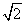instead of 1.414, which is only an approximation to three decimal places.

Other Roots

Above, we discussed square roots exclusively, and we noted that the radical symbol by itself indicates the square root. In some instances, however, we might be interested in calculating other roots of numbers. To reiterate, the square root of n is a number r, where the following relations apply.We can also calculate, for instance, the third root (also called the cube root) of a number n. To represent the third root, we add a small number 3 next to the radical as shown in the relations below. These expressions relate the number n and its cube root r.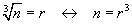Let's take a look at a few examples.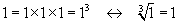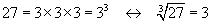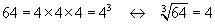We can also define a general kth root of a number n; that is,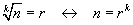In other words, if some number n is equal to a number r raised to the power of k, then the kth root of n is r. Some further examples are shown below.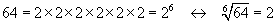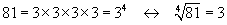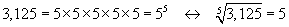Calculating roots beyond the square root mentally or by hand gets increasingly difficult; thus, in such cases, a calculator is generally needed. Furthermore, very few numbers have kth roots that are integers, meaning that most kth roots are irrational numbers, which cannot be written exactly in decimal or fractional form. We will deal primarily with square roots; you should now, however, have some familiarity with other roots. We now also have a sufficient foundation to allow us to relate roots to exponents.

Practice Problem: Evaluate the expression in each case.

a.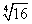b.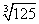c.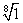Solution: As with the previous practice problem, these numbers do not require you to use a calculator, but they may require some careful thought (and perhaps some trial and error). In part c, note that 1 raised to any exponent (expressed as c) is 1.

a.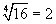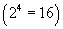b.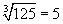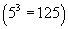c.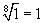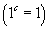Fractional Exponents

Before considering some rules for dealing with radicals, we can learn much about them just by relating them to exponents. Note that we used exponents in explaining the meaning of a root (and the radical symbol):We can apply the rules of exponents to the second expression,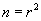. Recall the rule shown below: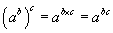Turning back to the expression, let's rewrite the left-hand side (n) using the above rule and making the assumption that the rule works for fractional exponents as well as integer exponents (we have no reason to assume otherwise at this point).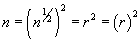Note that the expressions in parentheses (r and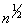) must be equal: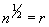Now, let's compare this result with the other relation from the beginning of this particular discussion: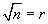Thus, a square root (radical) is the same as a fractional exponent-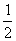, in this case. We can use similar reasoning to show that the kth root of a number n is the same as n raised to the power of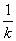.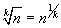Thus, we can approach radical expressions on their own terms or as exponential expressions. In some cases, writing the expression using exponents can simplify the math; in other cases, sticking with the radical form is better. In any event, we have now described the meaning of all exponents, including both positive and negative values as well as integer and fractional values. In addition, we can use the rules for a product or quotient raised to a power to show how the radical is distributed in such cases.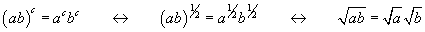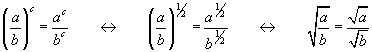Practice Problem: Evaluate each of the following expressions.

a.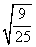b.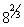c.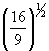Solution: In each case, apply the rules of radicals (or exponents) to evaluate the expression. In part b, we can divide the exponent into a product of an integer (2) and a fraction (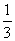) to simplify calculation of the result.

a.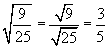b.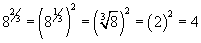c.